# 3 Quick Ways to Copy Down Excel Formula without IncrementingWhile working in Excel, we often have to copy down one formula to another group of cells without incrementing. Today I will be showing three easy ways to execute this.

## How to Copy Down Excel Formula without Incrementing

Let us have a look at this data set. We have the price record of various items of a company named APEX Garments.

There are the Item Names, their prices, tax, and prices with tax in columns B, C, D, and E respectively.

In the first cell of column E, prices with tax,we have written a formula

`=C4+C4*D4`Now we want to copy down this formula to the rest of the cells without incrementing the tax, D4.

That means the cell E5 will have:

`=C5+C5*D4`

Cell E6 will have:

`=C6+C6*D4`

And so on.

How can you achieve that?

Here are the methods you can utilize.

### 1.  Using Absolute Cell Reference

The best way to achieve this is using Absolute Cell Reference.

An Absolute Cell Reference is a cell reference having Dollar Sign(\$) before the row and column number.

When we drag a formula with an Absolute Cell Reference through the Fill Handle, it does not increment.

The Absolute Cell Reference of cell D4 is \$D\$4.

So use this formula in the Formula Bar for cell E4

`=C4+C4*\$D\$4`For Excel version 2013 or higher, you can use your keyboard shortcut to create an Absolute Cell Reference.

• Double click in the Formula Bar or press F2 on your keyboard. The formula will be on Edit mode.
• Put the cursor after D4 and press F4 on your keyboard. It will turn D4 into \$D\$4.
• If you press F4 again, it will turn \$D\$4 into D\$4.
• Press F4 again and you will get \$D4.
• If you press F4 again, you will get D4.
• Again press F4, you will get \$D\$4. And the cycle goes on.
• If your formula contains more than one cell reference and you need to make all of them Absolute, press Ctrl + Shift + Home first. It will select the whole formula. Then press F4.
• In the Formula Bar, the mouse cursor remains at the end by default. If it is not, you can press Ctrl + End on your keyboard to bring it to the end.

After entering the formula of the first cell with Absolute Cell Reference in the Formula Bar,

You have to copy the formula to the rest of the cells.

You can implement this in two methods.

#### Method 1: By Dragging the Fill Handle

Drag the Fill Handle (The Small Plus(+) Sign in the Bottom Right Corner) from the cell having formula with Absolute Cell Reference up to the cell in which you want to copy the formula.Here I drag the Fill Handle from cell E4 to E13.

And get the formula copied to all the cells without incrementing D4.#### Method 2: Using Fill Option from Excel Toolbar

• Select the cell with the formula having the Absolute Cell Reference and the rest of the cells where you want to copy down the formula. I select cells E4 to E13.• Then go to Home>Fill Option in Excel Toolbar under the Editing section.• Click on the drop down menu. You will get a few options. Click on Down.• You will get the formula copied to all cells without incrementing cell reference D4.### 2. Using the Find and Replace Box

This method is very handy when you want to copy formulas from one range of cells to another range of cells without changing the cell reference.

Let us think that we want to copy column E, price with tax to column F, with keeping all the formula intact. How can we do that?

•  Go to Home>Find and Select Option from Excel Toolbar.• Click on the Drop Down Menu. You will get some options. Select Replace.• You will get the Find and Replace dialogue box. You can also press Ctrl + H to get that.  In Find What option, insert ‘=’. And in Replace With option, insert ‘&&&’• Click on Replace All. You will get all cells in column E having ‘&&&’ like this.• Then select all cells of column E, copy them with Ctrl +C and then paste them in column F.• Again go to Home>Find and Select. Then select  Replace. (Or press Ctrl + H) This time, in the Find What option, insert ‘&&&’. And in the Replace With option, insert ‘=’.• Click on Replace All. You will find the formulas from column E copied to column F without any change.### 3. By creating a Macros (VBA)

You can use VBA code to create a Macros to do the same thing as I did earlier. Copy formulas from one column to another column.

• Press Alt + F11 in your Excel File. It will open the VBA window.
• Then go to the Insert option in the VBA Toolbar. Select Module.• You will get a Module window like this.• Write down the following code here to create the Macros.

Code

``````Sub PasteExactFormulas()
Dim vArr As Variant
Dim sRng As Range, rRng As Range, r As Long, c As Long
Set sRng = Application.InputBox("Select source Range w/formulas", Type:=8)
r = sRng.Rows.Count
c = sRng.Columns.Count
vArr = sRng.Formula
For i = 1 To UBound(vArr, 1)
For j = 1 To UBound(vArr, 2)
vArr(i, j) = CStr(vArr(i, j))
Next j
Next i
Set rRng = Application.InputBox("Select the first cell of paste range", Type:=8)
Set rRng = rRng.Resize(r, c)
rRng.Value = vArr
End Sub``````

This site helped us understand and develop the code.

• Your code will look like this in the module window.• Press Ctrl + C to save the Macros. You will get an Error Box like this.

##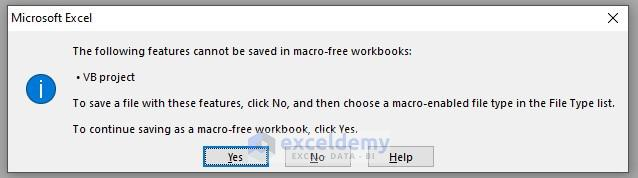• Click on No. Excel will automatically open the Save As window for you. Give the File Name anything. Then click on the drop down menu with Save As Type.

##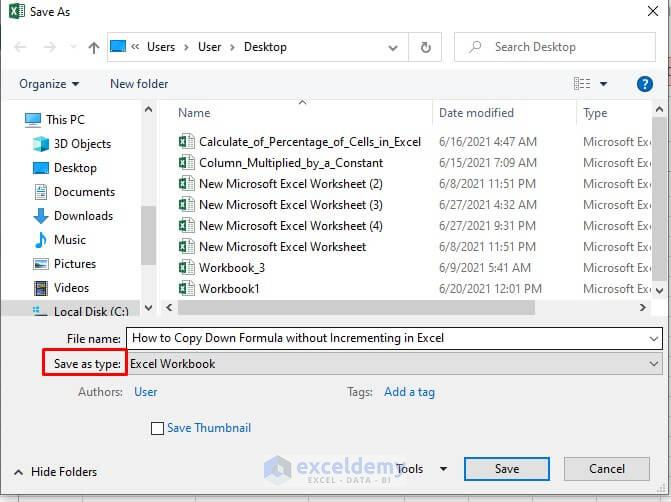• You will find a lot of options. Choose Excel-Macro-Enabled Workbook. Then click Save. Your workbook is now saved with the Macros.

##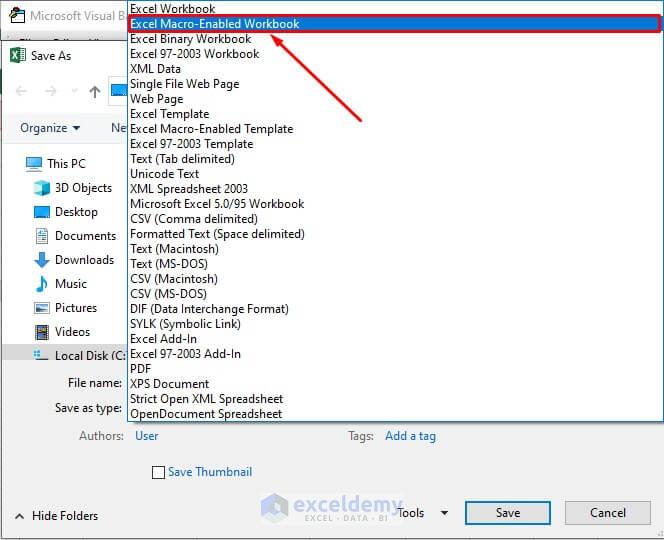• Then go back to the Excel Worksheet and press Alt + F8. You will get a box named Macros. Select the Macro you want to run, and click Run. Here I want to run PasteExactFormulas.

##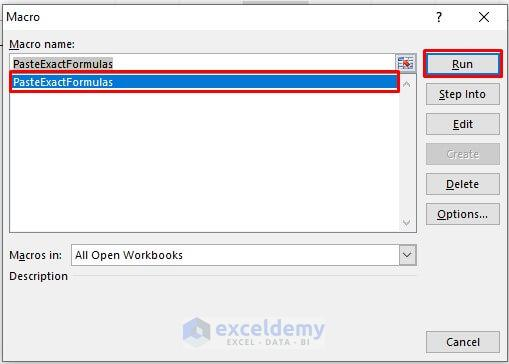• If you run the recently created Macro, the PasteExactFormulas, you will get a Input Box like this. Select the range of the cells from which you want to copy the formulas. Then click OK. Here I select cells E3 to E13.

##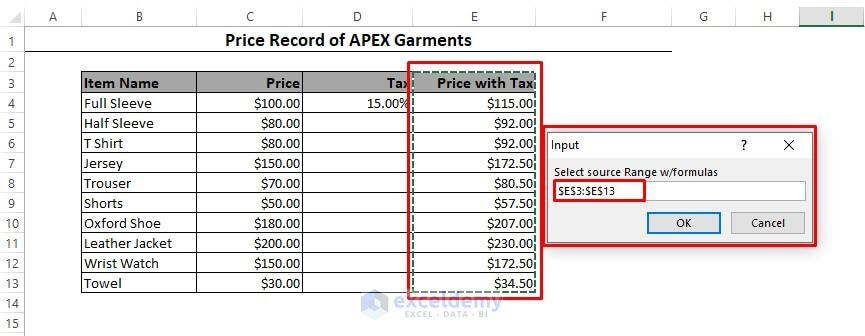• You will get another Input Box like this. Select the first cell of the range where you want to paste the formulas. Then click OK. Here I select F3.

##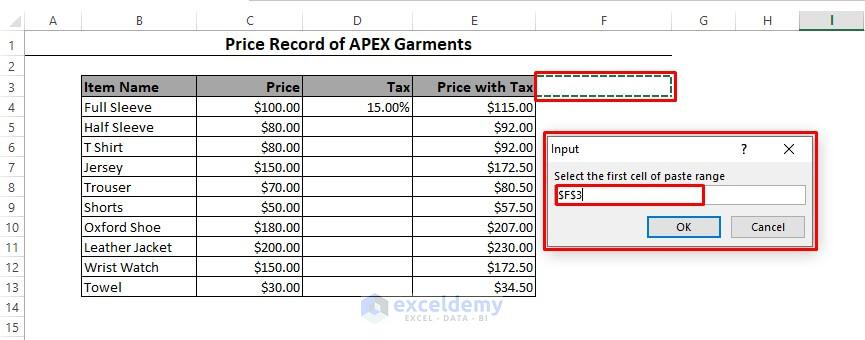• And you will find the formulas of column E copied beautifully to column F. Obviously this does not copy the format of the cells, only the formula. If you want, you can change the format manually.

## Conclusion

Using these methods you can copy down formulas in Excel without any increment. These are pretty convenient. Do you know any other method? Let us know in the comment section.1. Reply